# Test Prep Plan - Take a practice test

Take this practice test to check your existing knowledge of the course material. We'll review your answers and create a Test Prep Plan for you based on your results.
How Test Prep Plans work
1
2Based on your results, we'll create a customized Test Prep Plan just for you!
3Study smarter
Study more effectively: skip concepts you already know and focus on what you still need to learn.

# High School Geometry: Properties of Triangles Chapter Exam

Exam Instructions:

Choose your answers to the questions and click 'Next' to see the next set of questions. You can skip questions if you would like and come back to them later with the yellow "Go To First Skipped Question" button. When you have completed the practice exam, a green submit button will appear. Click it to see your results. Good luck!

### Page 1

#### Question 1 1. In the pictured triangle, what is the centroid?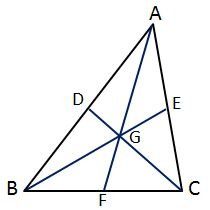#### Question 2 2. What type of triangle is pictured?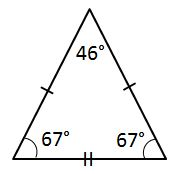#### Question 3 3. What type of triangle is pictured?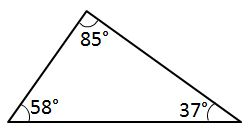#### Question 4 4. What type of triangle is pictured?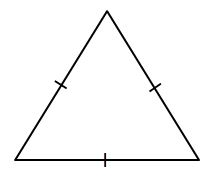#### Question 5 5. Determine if triangle VEZ is similar to triangle MAG.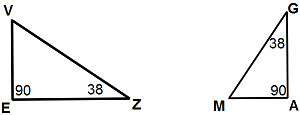### Page 2

#### Question 9 9. What type of line segment is represented by the dotted line in the pictured triangle?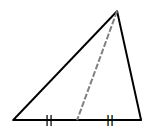#### Question 10 10. Which statement is true regarding areas based on the pictured triangle? Point G is the centroid.### Page 3

#### Question 11 11. What type of line segment is represented by the dotted line in the pictured triangle?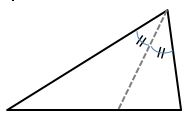#### Question 12 12. In the figure below, Angle 13 = 10y and Angle 11 = 3y + 11. Determine the measure of Angle 16.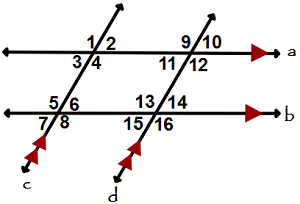#### Question 13 13. If angle a is 60 degrees, what is the measure of angle b?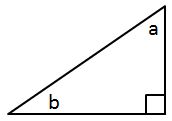### Page 4

#### Question 17 17. If angle a is 68 degrees and angle b is 37 degrees, what is the measure of angle c?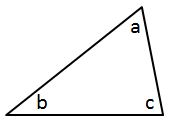#### Question 19 19. Given the triangle PQE, calculate the measure of Angle Q.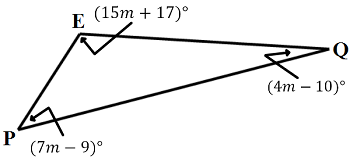#### Question 20 20. If angle a is 80 degrees, what is the measure of angle d?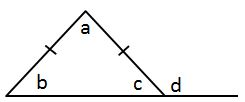### Page 5

#### Question 23 23. In triangle GBN, Angle G is seven more than three times a number, Angle B is five more than four times the number, and Angle N is twelve less than five times the number. Solve for the measure of Angle G.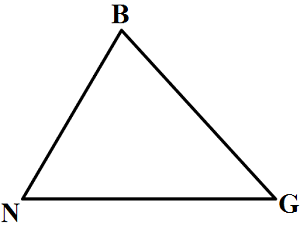#### Question 24 24. If angle a is 30 degrees and angle d is 70 degrees, what is the measure of angle b?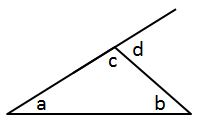#### Question 25 25. In the pictured triangle, identify the altitude(s).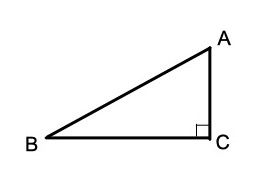### Page 6

#### Question 26 26. What type of line segment is represented by the vertical dotted line in the pictured triangle?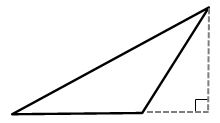#### Question 27 27. Mary claims that the triangles below are similar by AA. Do you agree or disagree with Mary?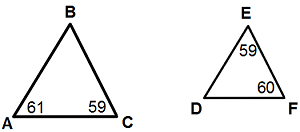#### Question 30 30. Is triangle ABC similar to triangle DEF?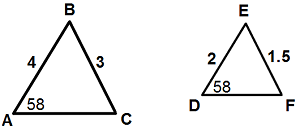#### High School Geometry: Properties of Triangles Chapter Exam Instructions

Choose your answers to the questions and click 'Next' to see the next set of questions. You can skip questions if you would like and come back to them later with the yellow "Go To First Skipped Question" button. When you have completed the practice exam, a green submit button will appear. Click it to see your results. Good luck!

Support